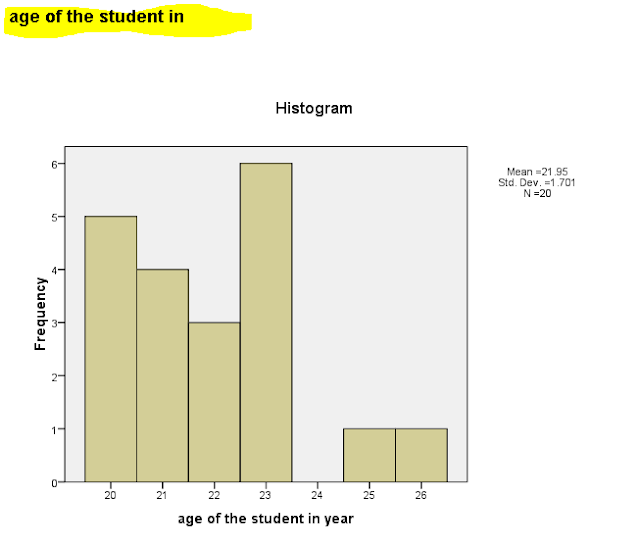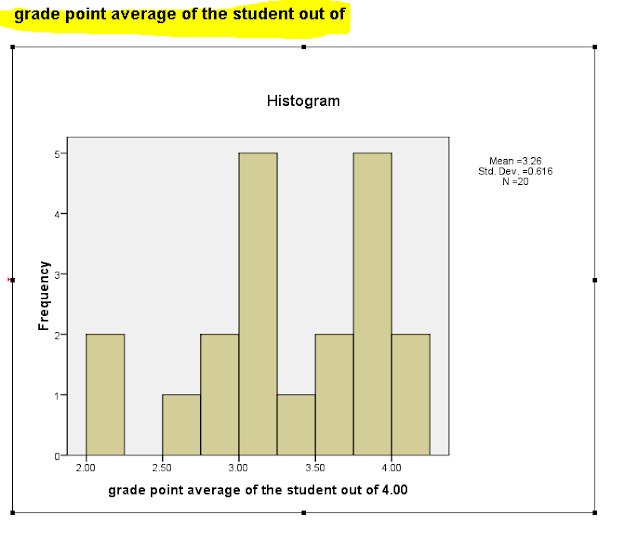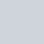# Normality checking of a data set using spss

Normality checking is a procedure of check the normality of our working data set. Basically we check the normality using histogram. If the shape of the histogram is bell shaped then the data set follow the normal distribution. We also use the normality table including kolmogrov-smirnov test and shapiro-wilk test value.

• If the test value or p-values are less than 0.05 then the test is significant and the data not follow the normal distribution.
• If the test value is equal or greater than 0.05 then the test is not significant and the data follow the normal distribution.

### Normality checking procedure using spss

 Normality check

1. we have the following data set–

 Data set

2. First we have to go to Analyze→→ Descriptive statistics →→Explore

 Explore

3. Drag the working variable into the Dependent list and click on Plot.

 Explore

4. Select Histogram and Normality plot with test and click on Continue.

 Select histogram & normality polt

5. click on OKClick on OK

6. Now we can see the output window.Test of normality table

From the above table we can see that the p-values for the first random variable is .139 and .029 respectively. Here one value is less than 0.05 so the first variable not follow the normal distribution.
And the second variable where p-vales are .200 and .106 respectively which are greater than .05, so the second variable follow the normal distribution.Histogram-1Histogram-2

### One thought on “Normality checking of a data set using spss”

1.Unknown

very effective for new researchers